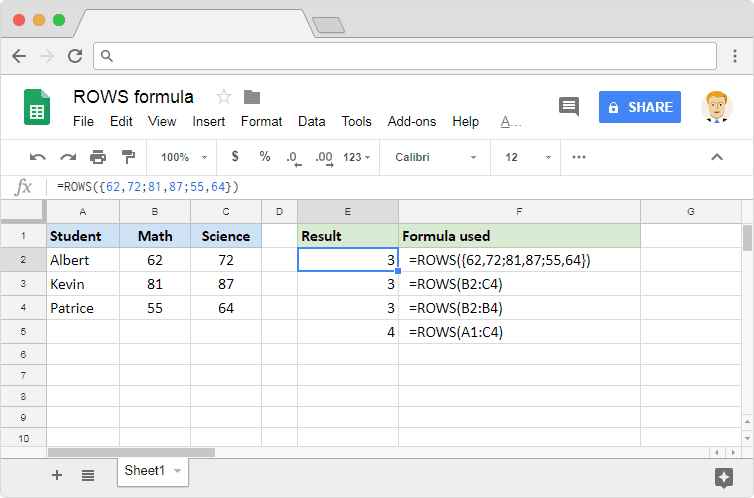Select Page

How to use the ROWS formula in Google Sheets

Jul 15, 2018

Assume that we have a range of cells in a spreadsheet that we are interested in. And we want to know how many rows it has spread across. We could probably do a quick count manually if there are only a few of them. But more often than not, we encounter instances where the rows in a range are more than just a few. Or, it could be that we need to dynamically generate the output, rather than resorting to manual counting methods. We can make use of the ROWS formula in Google Sheets for such situations.

Syntax

ROWS(range)

• range – is the range of values, or the address reference to the range of cells, whose row count the formula returns as output.

Usage: ROWS formula in Google Sheets

On the face of it, the syntax may look pretty obvious and simple. But it helps to enhance our understanding with the help of a few practical examples. So, let us get straight into applying the formula on Google Sheets application. Consider the screenshot below.For us to be able to input values directly as a range, we need to use a pair of curly brackets. We demonstrated this case in the first example. Is there a way to separate this range of direct values into columns and rows? Yes, and it is actually very simple. We use commas to separate the values into columns, and a semi-colon to separate them into rows. Please observe the first example closely. The range {62,72;81,87;55,64} consists of three rows and two columns. Therefore the formula returned the output as 3.

In the second example, we used the reference to the range of cells instead of inputting the values directly. The range B2:C4 also spans across three rows and two columns. Hence the formula evaluates the output to 3, just like it did in the first example.

Regardless of the number of columns within the range, the ROWS formula returns only the number of rows within that range. The range B2:B4 used in the third example has just one column, but that doesn’t impact the output. That is because there are three rows from 2 through 4.

The fourth example shows just another range, that consists of four rows. Hence the row formula returns 4 as the output.

Questions or remarks?

If you have any questions or remarks on this post, please visit our community and do not hesitate to contact us there! Join

What is Sheetgo?

Sheetgo is a cloud-based software that allows you to create and automate workflows straight from your spreadsheet. Try itDon't hesitate to reach us anytime! support@sheetgo.com

Resources

© 2016-2019 SHEETGO EUROPE SL